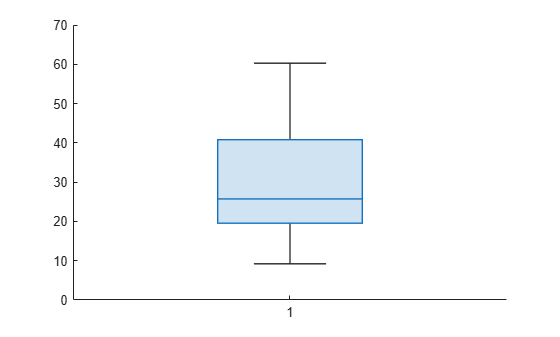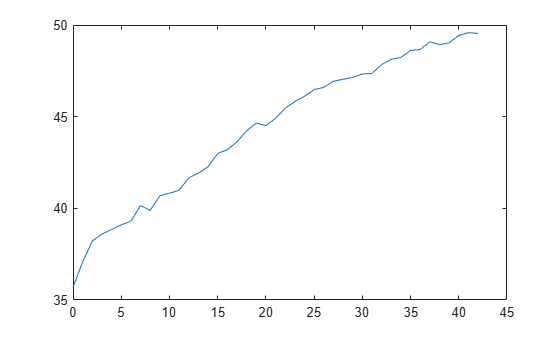kfoldLoss

Loss for cross-validated partitioned regression model

Description

example

L = kfoldLoss(CVMdl) returns the loss (mean squared error) obtained by the cross-validated regression model CVMdl. For every fold, kfoldLoss computes the loss for validation-fold observations using a model trained on training-fold observations. CVMdl.X and CVMdl.Y contain both sets of observations.

example

L = kfoldLoss(CVMdl,Name,Value) returns the loss with additional options specified by one or more name-value arguments. For example, you can specify a custom loss function.

Examples

collapse all

Find the cross-validation loss for a regression ensemble of the carsmall data.

Load the carsmall data set and select displacement, horsepower, and vehicle weight as predictors.

X = [Displacement Horsepower Weight];

Train an ensemble of regression trees.

rens = fitrensemble(X,MPG);

Create a cross-validated ensemble from rens and find the k-fold cross-validation loss.

rng(10,'twister') % For reproducibility
cvrens = crossval(rens);
L = kfoldLoss(cvrens)
L = 28.7114

The mean squared error (MSE) is a measure of model quality. Examine the MSE for each fold of a cross-validated regression model.

Load the carsmall data set. Specify the predictor X and the response data Y.

X = [Cylinders Displacement Horsepower Weight];
Y = MPG;

Train a cross-validated regression tree model. By default, the software implements 10-fold cross-validation.

rng('default') % For reproducibility
CVMdl = fitrtree(X,Y,'CrossVal','on');

Compute the MSE for each fold. Visualize the distribution of the loss values by using a box plot. Notice that none of the values is an outlier.

losses = kfoldLoss(CVMdl,'Mode','individual')
losses = 10×1

42.5072
20.3995
22.3737
34.4255
40.8005
60.2755
19.5562
9.2060
29.0788
16.3386

boxchart(losses)Train a cross-validated generalized additive model (GAM) with 10 folds. Then, use kfoldLoss to compute the cumulative cross-validation regression loss (mean squared errors). Use the errors to determine the optimal number of trees per predictor (linear term for predictor) and the optimal number of trees per interaction term.

Alternatively, you can find optimal values of fitrgam name-value arguments by using the OptimizeHyperparameters name-value argument. For an example, see Optimize GAM Using OptimizeHyperparameters.

Create a table that contains the predictor variables (Age, Diastolic, Smoker, Weight, Gender, and SelfAssessedHealthStatus) and the response variable (Systolic).

tbl = table(Age,Diastolic,Smoker,Weight,Gender,SelfAssessedHealthStatus,Systolic);

Create a cross-validated GAM by using the default cross-validation option. Specify the 'CrossVal' name-value argument as 'on'. Also, specify to include 5 interaction terms.

rng('default') % For reproducibility
CVMdl = fitrgam(tbl,'Systolic','CrossVal','on','Interactions',5);

If you specify 'Mode' as 'cumulative' for kfoldLoss, then the function returns cumulative errors, which are the average errors across all folds obtained using the same number of trees for each fold. Display the number of trees for each fold.

CVMdl.NumTrainedPerFold
ans = struct with fields:
PredictorTrees: [300 300 300 300 300 300 300 300 300 300]
InteractionTrees: [76 100 100 100 100 42 100 100 59 100]

kfoldLoss can compute cumulative errors using up to 300 predictor trees and 42 interaction trees.

Plot the cumulative, 10-fold cross-validated, mean squared errors. Specify 'IncludeInteractions' as false to exclude interaction terms from the computation.

L_noInteractions = kfoldLoss(CVMdl,'Mode','cumulative','IncludeInteractions',false);
figure
plot(0:min(CVMdl.NumTrainedPerFold.PredictorTrees),L_noInteractions)The first element of L_noInteractions is the average error over all folds obtained using only the intercept (constant) term. The (J+1)th element of L_noInteractions is the average error obtained using the intercept term and the first J predictor trees per linear term. Plotting the cumulative loss allows you to monitor how the error changes as the number of predictor trees in the GAM increases.

Find the minimum error and the number of predictor trees used to achieve the minimum error.

[M,I] = min(L_noInteractions)
M = 28.0506

I = 6

The GAM achieves the minimum error when it includes 5 predictor trees.

Compute the cumulative mean squared error using both linear terms and interaction terms.

L = kfoldLoss(CVMdl,'Mode','cumulative');
figure
plot(0:min(CVMdl.NumTrainedPerFold.InteractionTrees),L)The first element of L is the average error over all folds obtained using the intercept (constant) term and all predictor trees per linear term. The (J+1)th element of L is the average error obtained using the intercept term, all predictor trees per linear term, and the first J interaction trees per interaction term. The plot shows that the error increases when interaction terms are added.

If you are satisfied with the error when the number of predictor trees is 5, you can create a predictive model by training the univariate GAM again and specifying 'NumTreesPerPredictor',5 without cross-validation.

Input Arguments

collapse all

Cross-validated partitioned regression model, specified as a RegressionPartitionedModel, RegressionPartitionedEnsemble, RegressionPartitionedGAM, or RegressionPartitionedSVM object. You can create the object in two ways:

• Pass a trained regression model listed in the following table to its crossval object function.

• Train a regression model using a function listed in the following table and specify one of the cross-validation name-value arguments for the function.

Name-Value Arguments

Specify optional comma-separated pairs of Name,Value arguments. Name is the argument name and Value is the corresponding value. Name must appear inside quotes. You can specify several name and value pair arguments in any order as Name1,Value1,...,NameN,ValueN.

Example: kfoldLoss(CVMdl,'Folds',[1 2 3 5]) specifies to use the first, second, third, and fifth folds to compute the mean squared error, but to exclude the fourth fold.

Fold indices to use, specified as a positive integer vector. The elements of Folds must be within the range from 1 to CVMdl.KFold.

The software uses only the folds specified in Folds.

Example: 'Folds',[1 4 10]

Data Types: single | double

Flag to include interaction terms of the model, specified as true or false. This argument is valid only for a generalized additive model (GAM). That is, you can specify this argument only when CVMdl is RegressionPartitionedGAM.

The default value is true if the models in CVMdl (CVMdl.Trained) contain interaction terms. The value must be false if the models do not contain interaction terms.

Example: 'IncludeInteractions',false

Data Types: logical

Loss function, specified as 'mse' or a function handle.

• Specify the built-in function 'mse'. In this case, the loss function is the mean squared error.

• Specify your own function using function handle notation.

Assume that n is the number of observations in the training data (CVMdl.NumObservations). Your function must have the signature lossvalue = lossfun(Y,Yfit,W), where:

• The output argument lossvalue is a scalar.

• You specify the function name (lossfun).

• Y is an n-by-1 numeric vector of observed responses.

• Yfit is an n-by-1 numeric vector of predicted responses.

• W is an n-by-1 numeric vector of observation weights.

Data Types: char | string | function_handle

Aggregation level for the output, specified as 'average', 'individual', or 'cumulative'.

ValueDescription
'average'The output is a scalar average over all folds.
'individual'The output is a vector of length k containing one value per fold, where k is the number of folds.
'cumulative'

Note

If you want to specify this value, CVMdl must be a RegressionPartitionedEnsemble object or RegressionPartitionedGAM object.

• If CVMdl is RegressionPartitionedEnsemble, then the output is a vector of length min(CVMdl.NumTrainedPerFold). Each element j is an average over all folds that the function obtains by using ensembles trained with weak learners 1:j.

• If CVMdl is RegressionPartitionedGAM, then the output value depends on the IncludeInteractions value.

• If IncludeInteractions is false, then L is a (1 + min(NumTrainedPerFold.PredictorTrees))-by-1 numeric column vector. The first element of L is an average over all folds that is obtained using only the intercept (constant) term. The (j + 1)th element of L is an average obtained using the intercept term and the first j predictor trees per linear term.

• If IncludeInteractions is true, then L is a (1 + min(NumTrainedPerFold.InteractionTrees))-by-1 numeric column vector. The first element of L is an average over all folds that is obtained using the intercept (constant) term and all predictor trees per linear term. The (j + 1)th element of L is an average obtained using the intercept term, all predictor trees per linear term, and the first j interaction trees per interaction term.

Example: 'Mode','individual'

Output Arguments

collapse all

Loss, returned as a numeric scalar or numeric column vector.

By default, the loss is the mean squared error between the validation-fold observations and the predictions made with a regression model trained on the training-fold observations.

• If Mode is 'average', then L is the average loss over all folds.

• If Mode is 'individual', then L is a k-by-1 numeric column vector containing the loss for each fold, where k is the number of folds.

• If Mode is 'cumulative' and CVMdl is RegressionPartitionedEnsemble, then L is a min(CVMdl.NumTrainedPerFold)-by-1 numeric column vector. Each element j is the average loss over all folds that the function obtains using ensembles trained with weak learners 1:j.

• If Mode is 'cumulative' and CVMdl is RegressionPartitionedGAM, then the output value depends on the IncludeInteractions value.

• If IncludeInteractions is false, then L is a (1 + min(NumTrainedPerFold.PredictorTrees))-by-1 numeric column vector. The first element of L is the average loss over all folds that is obtained using only the intercept (constant) term. The (j + 1)th element of L is the average loss obtained using the intercept term and the first j predictor trees per linear term.

• If IncludeInteractions is true, then L is a (1 + min(NumTrainedPerFold.InteractionTrees))-by-1 numeric column vector. The first element of L is the average loss over all folds that is obtained using the intercept (constant) term and all predictor trees per linear term. The (j + 1)th element of L is the average loss obtained using the intercept term, all predictor trees per linear term, and the first j interaction trees per interaction term.

Alternative Functionality

If you want to compute the cross-validated loss of a tree model, you can avoid constructing a RegressionPartitionedModel object by calling cvloss. Creating a cross-validated tree object can save you time if you plan to examine it more than once.

Extended Capabilities

Introduced in R2011a